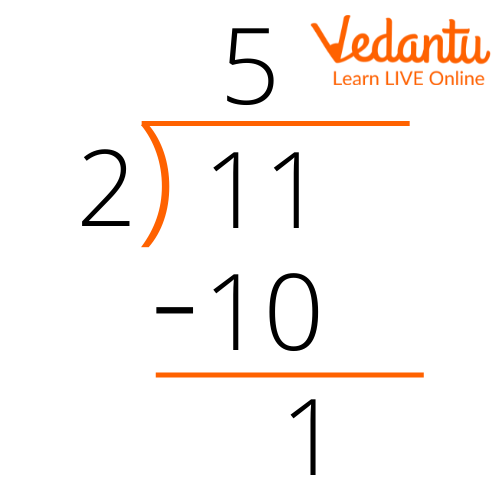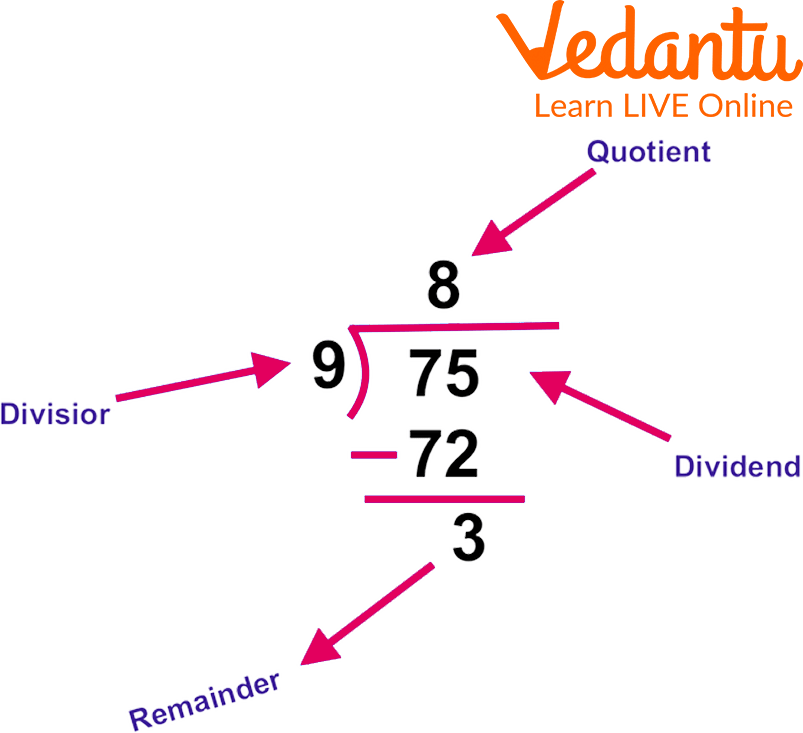Courses
Courses for Kids
Free study material
Free LIVE classes
More

# Concept of Divisor, Dividend, Quotient and RemainderLIVE
Join Vedantu’s FREE Mastercalss

## Understanding Divisor, Dividend, Quotient and Remainder in Maths

The dividend-quotient formula shows the relationship between the dividend, the divisor, the quotient and the remainder, which is one of the main aspects of division. Division is the process of dividing a number into equal parts, leaving a remainder if the number cannot be further divided. The quotient formula of the remainder of division is an important rule in division. Let us learn more about these concepts ahead!Parts of a Division

## What Is the Divisor Dividend Quotient Remainder?

• Divisor

A divisor is a number that divides the other number in the calculation. The divisor definition states that it is the term performing the division operation on the dividend. For example, when we divide the number 28 by the number 7, 7 is called the divisor, whereas the number 28 is called the dividend.

The formula for Divisor = (Dividend - remainder) ÷ Quotient

• Dividend

A dividend is a whole number or the number of things that need to be divided into certain equal parts. Dividend is the number that is to be divided by the divisor.

The formula for Dividend = Divisor x Quotient + Remainder

• Quotient

When you divide two numbers, the result of their division, called a quotient, will be a whole number. If the two numbers have no remainder when divided, they are called "perfect" factors and their quotient is their "product".

The formula for Quotient = Dividend ÷ Divisor.

• Remainder

In Mathematics, the remainder is the amount "left over" after performing some computation. In arithmetic, the remainder is the integer "left over" after dividing one integer by another to produce an integer quotient.

The formula for Remainder = dividend - (divisor × quotient)

For example, 75 divided by 9 gives 8 as a quotient and 3 as a remainder.Divisor Dividend Quotient and Remainder

## Where Are Dividend, Divisor, Quotient and Remainder Used in Maths?

The divisor is used in the decimal system to convert a decimal number into fractions. The quotient is used in the multiplication of two whole numbers: for example, 4×5=20, which was simplified from $4 \times 5=20\Rightarrow \dfrac{(4 \times 10)}{2}$.

## Solved Examples

Q 1 Divide 217 by 4.

Ans: $\dfrac{217}{4}$

Here, Dividend = 217

Divisor = 4

Quotient = 54

Remainder = 1

Q 2 Find the remainder when the dividend is 75, the divisor is 5 and the quotient is 15.

Ans: Given, dividend = 75, divisor = 5, quotient = 15 and let the remainder be x

75 = 5 × 15 + x

75 = 75 + x

x = 75 - 75

x = 0

Therefore, by using the formula we obtained the remainder which is 0. Remainder = 0

Q 3 Find the remainder when the dividend is 63, the divisor is 2 and the quotient is 31.

Ans: Given, dividend = 63, divisor = 2, quotient = 31 and let the remainder be x

63 = 2 × 31 + x

63 = 62 + x

x = 63 - 62

x = 1

Q 4 Divide 5679 by 7.

Ans: $\dfrac{5679}{7}$

Here, Dividend = 5679

Divisor = 7

Quotient = 811

Remainder = 2

Q 5 Find the remainder when the dividend is 57, the divisor is 8 and the quotient is 7.

Ans: Given, dividend = 75, divisor = 5, quotient = 15 and let the remainder be x

57 = 8 × 7 + x

57 = 56 + x

x = 57 -56

x = 1

Therefore, by using the formula we obtained the remainder which is 1. Remainder =1

## Practice Questions

Q 1 Divide 120 by 5 and find the quotient and the remainder.

Ans: Quotient = 24

Remainder = 0

Q 2 Find the dividend when the remainder is 1, the divisor is 3, and the quotient is 31.

Ans: Dividend = 94

Q 3 Find the remainder when the dividend is 55, the divisor is 3 and the quotient is 18.

Ans: Remainder = 1

## Summary

In conclusion, the number which is getting divided here is called the dividend. The number which divides a given number is the divisor. Also, the number which we get as a result is known as the quotient. The divisor which does not divide a number completely produces a number, which is referred to as remainder. We now hope you have a clear understanding of divisor, dividend, quotient and remainder.

Last updated date: 28th Sep 2023
Total views: 111.6k
Views today: 2.11k

## FAQs on Concept of Divisor, Dividend, Quotient and Remainder

1. Why is it important to learn multiplication and division?

Learning multiplication and division builds confidence in children to solve mathematical problems. The following are two primary reasons to do so:

• A child's confidence in the subject will increase if they can perform this operation with fluency and quick recall.

• This fundamental ability is needed for many of the jobs they are expected to complete at home and at school.

2. What is the use of the divisor, dividend, quotient, and remainder formula?

The dividend, divisor, and quotient formula consists of 4 main aspects used in division i.e. dividend, divisor, quotient, and remainder.

• A dividend is a number that is divided by the divisor.

• The divisor is the factor that divides the dividend. This formula is used for division. It makes the process of division easy.

3. In the formulae for divisor, dividend, quotient, and remainder, what happens if zero is used?

There are three possibilities when zero is used in the dividend divisor quotient remainder formula:

• A number divided by zero has a quotient of zero

• There is no way to divide a number by zero

• Dividends are entirely divisible by divisors, so there will be no remainder